paulgorman.org

# Electronics notes

## Basics

• Things can hold a positive or negative charge. (They can also be neutral.)
• Similar chages repel (positive repels positive, negative repels negative).
• Opposite charges attract (negative attracts positive, and visa versa).
• Electricity moves from negatively charged things to positively charged things.
• Voltage is the difference in charge (electrons) between two points. The greater the difference, the more the charge wants to move (more potential energy).
• The movement of electrons is called current, and is measures in amperes (amps).
• The amount of work done (power) by the voltage (V) and current (I) is measured in watts. P = VI
• Current moving through a wire creates a magnetic "wake".
• Magnetic fields generated by current in one wire can induce current in an adjacent wire. That induction in the second wire moves current in the opposite direction of the current in the first wire. The current in the second wire will in turn generate its own magnetic field.
• Current can cause self-induction in one and the same wire through which it flows, most significantly when current is starting or stopping.

## The water analogy for voltage, current, and resistance

Imagine a tank full of water, with a hole or outlet near the bottom.

The greater the volume of water in the tank, the greater the water pressure. This is like voltage, which is a measure of electric pressure.

The diameter of the hole or outlet at the bottom of the tank is like the resistance of an electrical circuit.

The rate at which water flows out of the tank is like the current in an electrical circuit, which is measured in amps.

## Charge

Charge is a force, much like gravity. The more charge something has, the more force it can exert. Charge is measured in Coulombs (abbreviated as "C").

There are two types of charge: positive and negative.

Charge is conserved, it can't be created or destroyed. However, charge can effectively be neutralized; equal positive charge and negative charge results in zero net charge. It is possible to cause neutral, apparently uncharged material to become unbalanced, charged. For example, rubbing a balloon on wool uses mechanical action to unbalance the balloon, creating a difference in charge (i.e.—stripping electrons from the balloon's atoms).

## Voltage

Force is the result of charge. Force attracts bodies of opposite charge. The magnitude of the force is proportional to the product of the charge on each mass. As with gravity, the term mass describes the quality of each body which results in such attraction. Also similar to gravity, the force between two objects has an inverse relationship to the distance between them—short distances mean strong forces.

It takes an opposing force to keep two bodies of opposite charge apart, just as you can hold a baseball in the air to prevent it from falling to the ground. To pull apart negative and positive charges apart requires work, like pulling a cart up a hill. The potential of separated charges for doing work (if they are released and allowed to fly together) is measured in Volts (abbreviated "V"). The greater the mass of the bodies, and the greater the distance, the greater the stored energy or Volts. Such energy is termed positive.

To compare the voltage of several set of bodies, start from a constant point called ground.

Just as two opposite bodies attract, two similarly charged bodies repel each other. Work can be done by letting such bodies fly apart. The voltage between two separated bodies of like charge is called negative, because they have already done work by flying apart and it would take external energy to force them together again.

## Current

Charge can move. Materials through each charge moves easily are called conductors, while materials which hamper the movement of charge are called insulators. If two oppositely charged bodies are connected by a conductor, electrical charged flows between them. This is called current. The current will continue until the two objects have exchanged enough positive and negative charge that they become balanced.

The amount of charge which in an electrical current which flows past a given point in one second is measured in amperes (abbreviated "a"). About one amp flows through a 100 watt light bulb. Digital circuits have much lower flow, measured in 1/1000 of an amp (a milliamp or ma) or even 1/1000 of a milliamp (a microamp or μa).

## BatteriesBatteries produce energy by using chemical reaction to separate charges to a positive or negative terminal. The voltage supplied by a battery depends upon the type of chemical reaction and the amount of chemicals in the battery. The current of the battery depends upon the speed of the chemical reaction and the surface area of the terminals.

## Resistors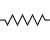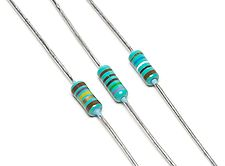Resistors limit the flow of current, like the flow of water from a pipe is limited by a valve. Resistors limit current by being poor conductors. The value of resistance is measured in Ohms (Ω). The colored bands on the resistor indicate its value.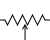There are various kinds of specialized resistors. A resistor with variable values is called a potentiometer. The resistance of a photoresistor or photocell decreases when exposed to light.

Multiple resistors will change the resistance.

Wired in a series, the total resistance is equal to the sum of the resistance of all resistors.

Wired in parallel, total resistance is calculated as follows:

RT = {1 {1R1} + {1R2}... + {1Rn}}

For example, if you have three resistors wired in parallel—two 8Ω resistors and one 4Ω resistor—the total resistance will be 2Ω (1/R = 1/8 + 1/8 + 1/4 = 1/2, so the resistance is 2).

Resistors wired in series provide more resistance than those wired in parallel.

### Ohm's law

Ohm's law describes the effect of voltage (V) and resistance (R) on current (I). High voltages and/or low resistances lead to large currents. As stated earlier, a resistor acts on electrical current the way a valve works on a water pipe. Ohm's law describes how and the voltage drops on one side of the resistor (the narrow side of the water valve).

V = IR or I = V/R

### Power

The current restriction of a resistor creates power (heat) in a manner similar to mechanical friction. Power is measured in Watts (W). Resistors can be damaged by heat, and most are rated to handle either 1/8, 1/4, 1, or 2 watts of power. The power across a resistor can be calculated as:

P = V2/R or P = I2R

## Capacitors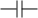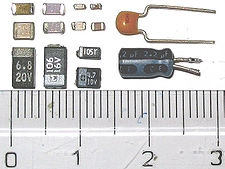Capacitors are made of two conductive plates—one positive and one negative— rolled up with an insulator to divide them. They function a bit like small batteries. They store energy to dampen the impact of rapid changes of voltage across a circuit, because it takes time for charge to flow into or out of the plates (particularly if a large resistor is opposing that flow). The ratio between the electrical charge on each plate vs the potential difference between the plates is measured in farads.

Wired in parallel, multiple capacitors result in a larger capacitance because more plates hold more voltage:

CT = C1 + C2... + Cn

However, when capacitors are wired in series, the capacitance of the series is lower than for any one of the individual capacitors, because the voltage is divided across the group—each plate holds less charge:

CT = {1 {1C1} + {1C2}... + {1Cn}}

Capacitors wired in parallel provide more capacitance than when wired in parallel.

## Inductors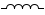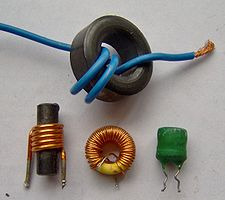Inductors are coils of wound wire. When current flows through any wire, a magnetic field forms. Running current through the coils of an inductor produces a significant magnetic field, and the mechanical energy of the field moving across the wire is induced into additional current. Increases or decreases in current take time to effect the magnetic field, so inductors act to smooth current variations much like capacitors smooth voltage variations.

Often a resistor or diode is used to prevent voltage damage to other components in the circuit from current leaving an inductor (if the power is switched off).

Inductors are measured in henries, millihenries, and microhenries.

As with capacitors, inductors wired in parallel increase inductance, but inductance is split between inductors when wired in series.

## Silicone and semiconductors

Pure silicone is not a conductor. Silicon doped with phosphorus, called n-type silicon, has extra electrons, which are not strongly attached. Doping silicon with boron (called p-type silicon) results in a vacancy of electrons, so that p-type silicon supports current.

## Diodes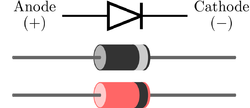A diode is one of the simplest semiconductor devices, and is comprised of two silicone sections: one doped p-type, the other n-type. Current can only flow between the two sections in one direction, like a one-way valve.

One common use of diodes is to rectify AC current into DC current, which is why diodes are sometimes called rectifiers (although there are other types of rectifiers).

## Using a multimeter

A multimeter can measure the voltage and resistance between two points on a circuit. Current is difficult to measure, but easy to calculate from the voltage and resistance using Ohm's law.